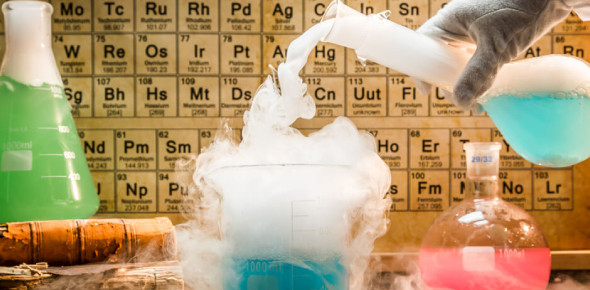# Chemical Reactions Quiz: MCQ Exam!

12 Questions | Attempts: 12409
ShareSettingsWhat do you know about chemical reactions? A chemical reaction is a route that leads to the transformation of one group of chemical substances to another. Chemical reactions exemplify changes that only involve electrons' positions in the developing and breaking of chemical bonds among atoms, with no change to the nuclei. This quiz will give you a positive reaction of happiness when you pass with flying colors.

• 1.
Which one of the following represents a physical change?
• A.

Iron rusting

• B.

Paper burning

• C.

Water freezing

• D.

Dynamite exploding

• 2.
.O {font-size:149%;} You can tell a chemical reaction because it always produces …
• A.

A different substance

• B.

Reactants

• C.

A change of state

• D.

Water

• 3.
Slide 5 To balance a chemical equation, you should change only the…
• A.

Subscripts

• B.

Coefficients

• C.

Chemical formulas

• D.

Location of the arrow

• 4.
Slide 6 .O {font-size:149%;} If iron combines with oxygen to make iron oxide, the reactants are…
• A.

Iron oxide

• B.

Iron and iron oxide

• C.

Iron and oxygen

• D.

Oxygen and iron oxide

• 5.
Slide 7 How many carbon atoms are there in  C12H22O11?
• A.

6

• B.

12

• C.

18

• D.

45

• 6.
Slide 8 How many oxygen atoms are in the product of this equation: 2H2 + O2 --> 2H2O
• A.

2

• B.

4

• C.

8

• D.

12

• 7.
Slide 9 .O {font-size:149%;} Which one of the following is a chemical equation?
• A.

Ice + heat --> water

• B.

Iron + oxygen --> rust

• C.

CO2

• D.

C + O2 --> CO2

• 8.
.O {font-size:149%;} Balance the following equation: Ag + O2 --> Ag2O
• A.

Put a 2 in front of Ag

• B.

Add a subscript 2 to the end of Ag2O

• C.

Add a 4 in front of Ag and a 2 in front of Ag2O

• D.

Add a 2 in front of Ag and a 1 in front of Ag2O

• 9.
.O {font-size:149%;} In a chemical equation, the arrow means what?
• A.

A reaction is happening.

• B.

The two sides are equal.

• C.

The products flow into reactants.

• D.

The equation is balanced.

• 10.
.O {font-size:149%;} The reactants include 7 grams of C and 4 grams of O. What is the total amount of C and O in the products?
• A.

7 grams

• B.

4 grams

• C.

11 grams

• D.

None

• 11.
.O {font-size:149%;} Who first determined that the masses of the reactants and products must be the same?
• A.

Pasteur

• B.

Archimedes

• C.

Galileo

• D.

Lavoisier

• 12.
.O {font-size:149%;} If you try to balance an equation by changing subscripts you change…
• A.

The number of molecules of product

• B.

The identity of the compound

• C.

The atomic number

• D.

The mass of the reactants

## Related TopicsBack to top
×

Wait!
Here's an interesting quiz for you.